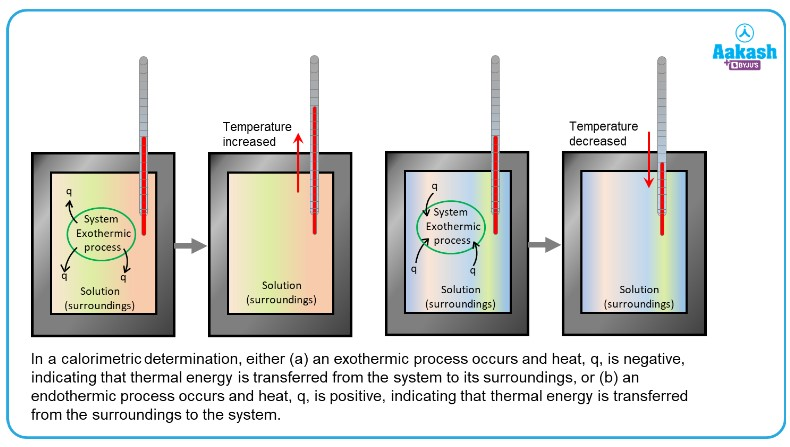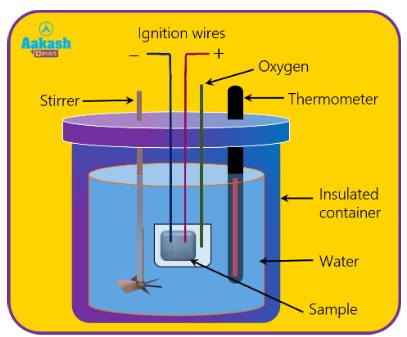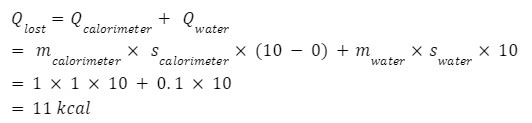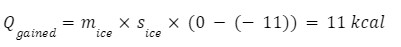• Call Now

1800-102-2727•

# Calorimeter - principle, construction, types and application, Solved Examples, FAQs

How do you measure heat involved in a process? One of the most commonly used techniques we can use to measure the amount of heat involved in a chemical or physical process is known as calorimetry. Calorimetry deals with the measurement of state of a body with respect to the thermal aspects in order to examine its chemical and physical changes.

## What is a Calorimeter?

A calorimeter is a device that is used for heat measurements which is necessary for calorimetry.

For example, when an exothermic reaction occurs in a solution in a given calorimeter, the heat produced during reaction is absorbed by the solution, which leads to rise in its temperature. Whereas, when an endothermic reaction occurs, the heat required is absorbed from the thermal energy of the solution, which further decreases its temperature(as shown in figure). The temperature change, along with the mass and specific heat of the solution, can then be used to calculate the amount of heat involved in either case.## Principle of calorimetry

Principle behind calorimetry is that in the case of an insulated system, the heat energy lost by a hot body is the same as the heat energy gained by the cold body. This heat transfer continues until both the bodies attain the same temperature.

The Calorimeter is based on the following formula.

We know that at steady state from the conservation of the energy,

Heat loss = Heat gain

q=mcΔt

Where q denotes the measure of the transfer of heat, m denotes the mass of substance, c denotes the specific heat capacity of the substance , Δt denotes the temperature change.

## Construction of calorimeter

It consists of a metallic vessel mainly made up of materials which are good conductors of electricity such as aluminum and copper. There is a setup for stirring the contents of the vessel. This metallic vessel with a stirrer is kept in an insulating jacket which helps in preventing the heat from escaping to the surrounding environment. It also has one opening through which we can insert a thermometer to measure the change in thermal properties inside the vessel.

The sample placed inside the vessel is burned with the help of ignition wires. To ensure that the heat loss by the sample sample is gained by water, the vessel is perfectly insulated to avoid any heat loss. The stirrer helps in uniform heating of water and the rise in temperature of water is measured using a thermometer. Thus using the rise in temperature, specific heat and mass, we can calculate the amount of heat released.## Water equivalent of calorimeter

The amount of water that is required to consume the same quantity of heat as that substance does for a temperature rise of one degree.

Water equivalent of a body, Water equivalent=Mass x specific heat

It can also be described as the mass of water whose heat capacity equals the heat capacity for the body.

Unit of water equivalent is kg and dimensional formula is [M].

## Types of Calorimeter and their applications

There are various types of calorimeters. Few of them are mentioned below.

• Reaction Calorimeter- Reaction calorimeter is used to measure the heat generated by the sensors in reactors.
• Adiabatic Calorimeter- Adiabatic calorimeters are commonly used to evaluate the runtime reactions.
• Bomb Calorimeter- Bomb calorimeters are often used to test the calorific value of solid and liquid fuels
• Differential Scanning Calorimeter- Differential scanning calorimeters is commonly used to find the change in any product's formula and its effects
• Constant Pressure Calorimeter- Constant pressure calorimeter measures the enthalpy change in both physical and chemical methods.

## Practice problems

Q 1. Calculate the amount of heat that is needed to change the temperature of 1 g of water by 40°C. Given that specific heat of water is 4.2 J/gm K.

Answer: C= 4.2 J/gm K; m= 1 g; ΔT= 40oC=40 K, then

According to equation Q= mCΔT,

Q= 1 4.2  x 40= 168 J

Q 2. 900 J of heat is applied to a mass of lead 0.5 kg to change its temperature from 20°C to 40°C. Determine its specific heat capacity.

Answer: Q= 900 J; m= 0.5 kg; ΔT= (40-20)°C= 20 °C,

S= 900/(0.5 x 20)

S= 90 J/kg K

Q 3. Calculate the heat capacity of a copper vessel of mass 160 g if the specific heat capacity of copper is 410 J/kg K.

Mass of copper vessel=160 g=0.16 kg

Heat Capacity= Mass X Specific heat capacity

=0.16 kg x 410 J/kg K

=65.6 J/K

Q 4. Water of mass m2= 1 kg is contained in a copper calorimeter of mass m1= 1 kg. Their common temperature is t=100C. Now a piece of ice of mass m3= 2 kg and temperature -110C is dropped into the calorimeter. Neglecting any heat loss, find the final temperature of the system.(specific heat of copper is 0.1 kcal/kg 0C, Specific heat of water is 1 kcal/kg0C, specific heat of ice is 0.5 kcal/kg0C, Latent heat of fusion of ice 78.7 kcal/kg 0C)

Heat lost = Heat GainedTherefore all heat lost by calorimeter and water is used in raising the temperature of ice from -110C to 00C .

Hence final temp of the system will be 00C, ice and water will coexist at 00C.

## FAQs

Q 1. Define 1 calorie?
A calorie is defined as the amount of heat required to raise the temperature of 1g of water from 14.5°C to 15.5°C at 760 mm Hg.

Q 2.State one application of bomb calorimeter?
A bomb calorimeter is used to determine the heat emitted from a given quantity of biomass sample combustion and to calculate the higher heating value (HHV) of that biomass fuel.

Q 3. Calorimeter is usually kept in a wooden box in order to insulate it thermally from the surroundings. What happens in its absence?
If the calorimeter is not kept in an insulated box then the heat of the calorimeter must be exchanged with the surrounding and hence violates the principle of calorimeter. As to determine the exact specific heat capacity, the total heat transferred must be known to us. So, a calorimeter is kept in a wooden box to insulate it thermally from the surroundings.

Q 4. Define the term calorimetry.
Measurement of the quantity of heat is known as calorimetry.